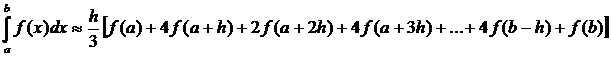«Актуальные вопросы в сфере социально-экономических, технических и естественных наук и информационных технологий» (3-4 апреля 2014г.)

Nechaiev D.Ya., Gnilenko A. B., Posudiievska O. R.

Oles Honchar Dnipropetrovsk National University

MODIFIED BESSEL FUNCTION OF THE FIRST SORT OF THE WHOLE ORDER

Today computers and programs are very important not only for everyday life, but also for the scientific world. The main problem of programming in science is simplification of computing process in research.

The matter of this work is: «Calculations of Bessel function of the first sort of the whole order»:For this purpose we need to write a dialogue for Windows application in a programming language C++ for function evaluation, using integral representations and expansions in a series. For data input we use an appendix with the corresponding dialog boxes for information input. For function evaluation in its integral representation it is worth using a numerical integration on Simpson’s method:,

where h – a step of splitting an interval (a, b).

Further it is necessary to calculate the value of function at certain interval of values of argument of  k . The display of the results is schedule which is removed to the separate MATLAB window, caused by the appendix.

After investigating the function on graphics we have to see the two curves: for the integral representation and for the expansion in a series.

Cylindrical functions arises in the time of finding out the solutions of the equation of Laplace and the equation of Helmholtz in cylindrical and spatial polar coordinates. Therefore cylindrical functions are applied in the solution of many problems of the wave propagation, static potentials, etc., for example:

– electromagnetic waves in a cylindrical wave guide;

– heat conductivity in cylindrical objects;

– forms of fluctuation of a thin round membrane;

– distribution of intensity of light diffracted on a hole;

– speed of particles in the cylinder filled with liquid and rotating round its pivot-center;

– wave functions in spherically symmetric potential box.

Cylindrical functions are also applied and in the solution of other tasks, for example, when processing signals. For these reasons it is worth investigating these functions by means of programming.

The list of references:

1. Electronic resource. – Mode of access: http://www.mathworld.wolfram.com